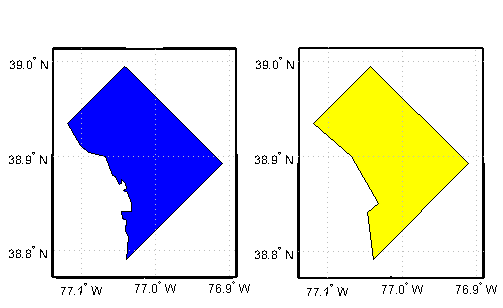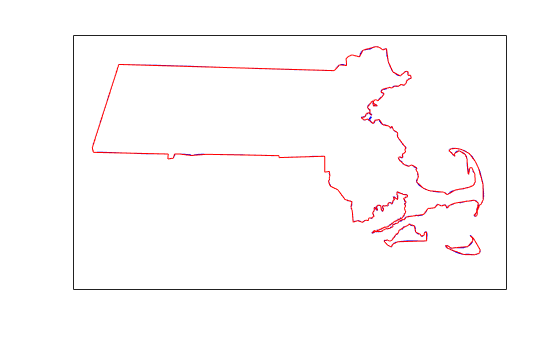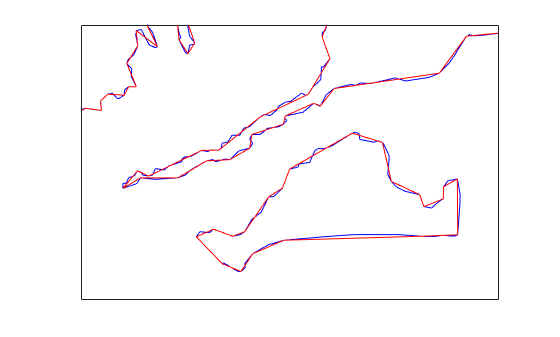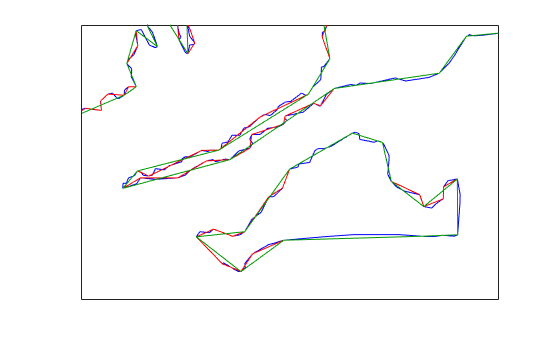# reducem

Reduce density of points in vector data

## Syntax

```[latout,lonout] = reducem(latin,lonin) [latout,lonout] = reducem(latin,lonin,tol) [latout,lonout,cerr] = reducem(...) [latout,lonout,cerr,tol] = reducem(...) ```

## Description

`[latout,lonout] = reducem(latin,lonin)` reduces the number of points in vector map data. In this case the tolerance is computed automatically.

`[latout,lonout] = reducem(latin,lonin,tol)` uses the provided tolerance. The units of the tolerance are degrees of arc on the surface of a sphere.

`[latout,lonout,cerr] = reducem(...)` in addition returns a measure of the error introduced by the simplification. The output `cerr` is the difference in the arc length of the original and reduced data, normalized by the original length.

`[latout,lonout,cerr,tol] = reducem(...)` also returns the tolerance used in the reduction, which is useful when the tolerance is computed automatically.

## Examples

collapse all

Compare the original and reduced borders of the District of Columbia from the shapefile `usastatehi.shp`.

Extract the borders of the District of Colombia.

```dc = shaperead('usastatehi.shp',... 'UseGeoCoords', true,... 'Selector',{@(name) ... strcmpi(name,'district of columbia'), 'Name'}); lat = extractfield(dc, 'Lat')'; lon = extractfield(dc, 'Lon')';```

Reduce the number of points in the border.

```[latreduced, lonreduced] = reducem(lat, lon); ```

Display the original and reduced borders.

```lonlim = dc.BoundingBox(:,1)' + [-0.02 0.02]; latlim = dc.BoundingBox(:,2)' + [-0.02 0.02]; subplot(1,2,1) usamap(latlim, lonlim); axis off geoshow(lat, lon,... 'DisplayType', 'polygon', 'FaceColor', 'blue') subplot(1,2,2) usamap(latlim, lonlim); axis off geoshow(latreduced, lonreduced,... 'DisplayType', 'polygon', 'FaceColor', 'yellow')```Simplifying polygon and line data can speed up certain calculations without making any noticeable impact on the data. One way to approach simplification is to use `reducem` with the default tolerance value and view the output. If the results do not meet your requirements, repeat the operation, increasing or decreasing the tolerance value to achieve the result you desire. The `reducem` function returns the tolerance value used.

Extract Massachusetts coastlines and state borders from the `usastatehi.shp` shapefile.

```ma = shaperead('usastatehi.shp','UseGeoCoords',true, ... 'Selector', {@(name)strcmpi(name,'Massachusetts'),'Name'}); masslat = ma.Lat; masslon = ma.Lon;```

The result is a pair of vectors that outline the state with 957 vertices.

`numel(masslat)`
```ans = 957 ```

Simplify Line Data Using Default Tolerance

Simplify the outline by using the `reducem` function with the default tolerance value.

`[masslat1, masslon1, cerr, tol] = reducem(masslat', masslon');`

Inspect the results. The number of vertices has been reduced to 252. The vectors have been reduced to about a quarter of their original lengths.

`numel(masslat1)`
```ans = 252 ```
`numel(masslat1)/numel(masslat)`
```ans = 0.2633 ```

Examine the error and tolerance values returned by `reducem`. The `cerr` value indicates that `reducem` has reduced the total length of the outline by about 3.3 percent. The tolerance that `reducem` used to achieve this reduction was 0.006 degrees, or about 660 meters.

`[cerr tol]`
```ans = 1×2 0.0331 0.0060 ```

Plot the reduced outline in red over the original outline in blue, using `geoshow`. At this resolution, it's hard to see any difference between the original outline and the reduced outline.

```figure axesm('MapProjection', 'eqdcyl', 'MapLatLim', [41.1 43.0],... 'MapLonLim', [-73.6, -69.8], 'Frame', 'off', 'Grid', 'off'); geoshow(masslat, masslon, 'DisplayType', 'line', 'color', 'blue') geoshow(masslat1, masslon1, 'DisplayType', 'line', 'color', 'red')```To get a better look at the two outlines, use `xlim` and `ylim` to zoom in on a portion of the map. Notice how the reduced outline conforms to the general contours of the original map but loses a lot of the detail.

```axesm('MapProjection', 'eqdcyl', 'MapLatLim', [41.1 43.0],... 'MapLonLim', [-73.6, -69.8], 'Frame', 'off', 'Grid', 'off'); xlim([0.0104 0.0198]) ylim([0.7202 0.7264]) geoshow(masslat, masslon, 'DisplayType', 'line', 'color', 'blue') geoshow(masslat1, masslon1, 'DisplayType', 'line', 'color', 'red')```Simplify Line Data Using Specified Tolerance

Perform the operation again, this time doubling the tolerance value.

```[masslat2,masslon2,cerr2,tol2] = reducem(masslat', masslon', 0.012); numel(masslat2)```
```ans = 157 ```
`numel(masslat2)/numel(masslat)`
```ans = 0.1641 ```

Examine the error and tolerance values returned by `reducem`. This time, the `cerr` value indicates that `reducem` has reduced the total length of the outline by about 5.2 percent. The tolerance that `reducem` used to achieve this reduction was 0.012 degrees.

`[cerr2 tol2]`
```ans = 1×2 0.0517 0.0120 ```

Plot this reduced outline in dark green over the original outline in blue. Note how this reduced outline maintains the general shape of the original map but loses much of the fine detail.

`geoshow(masslat2, masslon2, 'DisplayType', 'line', 'color', [0 .6 0])`## Tips

Vector data is reduced using the Douglas-Peucker line simplification algorithm. This method recursively subdivides a polygon until a run of points can be replaced by a straight line segment, with no point in that run deviating from the straight line by more than the tolerance. The distances used to decide on which runs of points to eliminate are computed in a Plate Carrée projection.

Reduced geographic data might not always be appropriate for display. If all intermediate points in a data set are reduced, then lines appearing straight in one projection are incorrectly displayed as straight lines in others.

## Version History

Introduced before R2006a